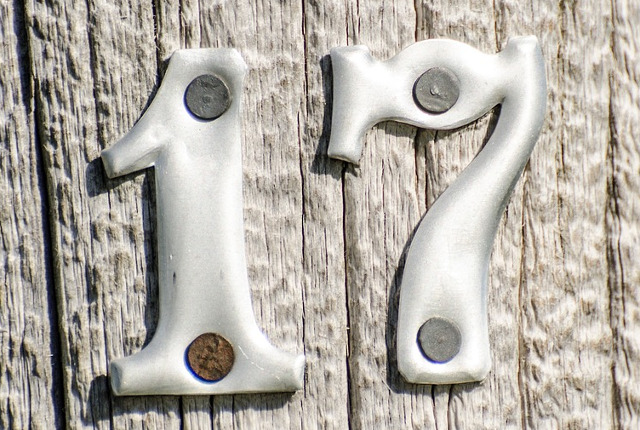# Compute absolute value of an int/float

yourbasic.org/golang## Integers

There is no built-in abs function for integers, but it’s simple to write your own.

``````// Abs returns the absolute value of x.
func Abs(x int64) int64 {
if x < 0 {
return -x
}
return x
}``````
Warning: The smallest value of a signed integer doesn’t have a matching positive value.
Unfortunately, our `Abs` function returns a negative value in this case.
``````fmt.Println(Abs(math.MinInt64))
// Output: -9223372036854775808
``````
(The Java and C libraries behave like this as well.)

## Floats

The `math.Abs` function returns the absolute value of `x`.

``func Abs(x float64) float64``

Special cases:

``````Abs(±Inf) = +Inf
Abs(NaN) = NaN``````

### More code examplesGo blueprints: code for com­mon tasks is a collection of handy code examples.